Your browser does not support JavaScript!

Search results

Some remarks on Zenga’s approach to kurtosis digital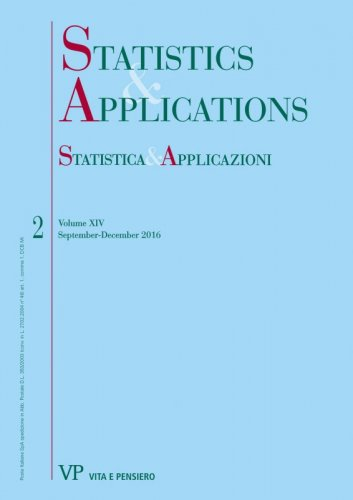format:Article| STATISTICA & APPLICAZIONI - 2016 - 2
Year: 2016
In this paper, several insights on the Zenga’s approach for the measurement of Kurtosis are provided. These insights mainly regard the connections between Kurtosis and Concentration indexes and the relation between the Kurtosis diagram and an extension of the well-known Lorenz curve, i.e. the relative first incomplete moment function...
The potential of the I(p) inequality curve in the analysis of empirical distributions digital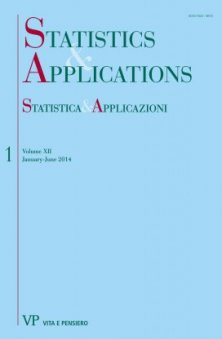format:Article| STATISTICA & APPLICAZIONI - 2014 - 1
Year: 2015
The possibility of analysing the inequality of a frequency distribution through a point measure is undoubtedly an advantage for any socioeconomic research. The Lorenz curve is the cornerstone on which other global inequality measures have been subsequently introduced...
A comparison among two generalized beta-mixtures of polisicchio distributions and the zenga model digital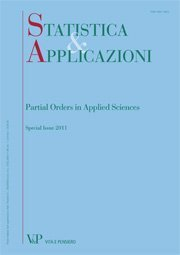format:Article| STATISTICA & APPLICAZIONI - 2013 - 2
Year: 2014
In this paper, the performances in fitting incomes of three different mixtures of Polisicchio distributions are compared...
The confluent hypergeometric-mixture of Polisicchio distributions: a generalized Zenga distribution
Free
digitalformat:Article| STATISTICA & APPLICAZIONI - 2013 - 1
Year: 2013
We propose a generalization of the three-parameters Zenga distribution obtaining a four-parameters model. The generalization is performed using the confluent hypergeometric distribution as mixing distributions in place of the classical beta. We compare the flexibility of the resulting model with that of the Zenga distribution observing some improvements.
More on M.M. Zenga’s new three-parameter distribution for nonnegative variables
Free
digital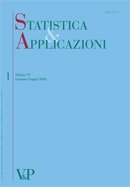format:Article| STATISTICA & APPLICAZIONI - 2011 - 1
Year: 2011
SUMMARY Recently Zenga (2010) has proposed a new three-parameter density function f (x : µ; α; θ), (µ > 0; α > 0; θ > 0), for non-negative variables. The parameter µ is equal to the expectation of the distribution. The new density has positive asymmetry and Paretian right tail. For θ > 1, Zenga (2010) has obtained the expressions of: the distribution function, the moments, the truncated moments, the mean deviation and Zenga’s (2007a) point inequality A(x) at x = µ. In the present paper, as to the general case θ > 0, the expressions of: the distribution function, the ordinary and truncated moments, the mean deviations and Zenga’s point inequality A (µ) are obtained. These expressions are more complex than those previously obtained for θ > 1 by Zenga (2010). The paper is enriched with many graphs of: the density functions (0.5 ≤ θ ≤ 1.5), the Lorenz L(p) and Zenga’s I (p) curves as well as the hazard and survival functions. Keywords: Non-Negative Variables, Positive Asymmetry, Paretian Right Tail, Beta Function, Lorenz Curve, Zenga’s Inequality Curve, Hazard Function, Survival Function.
Mixture of Polisicchio’s truncated Pareto distributions with beta weights
Free
digitalformat:Article| STATISTICA & APPLICAZIONI - 2010 - 1
Year: 2010
A new three-parameter density function f ðx : ; ; Þ for non-negative variables, obtained as a mixture of ‘‘Polisicchio’s truncated Pareto distributions’’, is proposed. The expectation of f ðx : ; ; Þ is equal to the parameter  > 0. The new density has positive asymmetry and Paretian right tail. The variance is equal to 2 3 ð þ 1Þ ½2 þ ð  1Þ   . The moments, the upper and lower truncated moments (with truncation at x ¼ ), are compact expressions of beta functions. Keywords: Income Distribution, Positive Asymmetry, Paretian Tail, Mixture Density, Beta Weights
The continuous random variable with uniform point inequality measure
Free
digitalformat:Article| STATISTICA & APPLICAZIONI - 2008 - 2
Year: 2008
By using the conditions that the expected value of an absolute random variable X is finite and positive and that the point inequality measure I ðpÞ is uniform for 0 < p < 1, this paper discusses the question of the existence of such random variable and proves that this problem has a unique solution. The obtained cumulative distribution function of X is a truncated Pareto distribution, with traditional inequality parameter equal to 0,5 and with support depending on the finite and positive expected value and the level of uniformity, based on the ratios between the lower means and the upper means, used for defining the point inequality measure I(p).
Using the properties of the arithmetic mean for least squares interpolation
Free
digitalformat:Article| STATISTICA & APPLICAZIONI - 2007 - 2
Year: 2007
In the present paper some properties of the arithmetic mean have been employed to determine the parameters of the least squares line, plane and hyper plane, without using partial derivatives and solving the equations of the normal system. It is in fact known that when the criterion of least squares is used to identify an interpolating function, the solution to the problem is obtained via the search for the minimum of a function; therefore the use of partial derivatives becomes inevitable. Instead, the method here suggested allows the interpolating model - which is common to many economic and social subjects - to be presented to university students including those without any knowledge of partial derivatives.
A few remarks on the estimation of some variability measures digital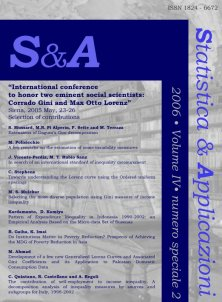format:Article| STATISTICA & APPLICAZIONI - 2006 - Special issue 2
Year: 2006
This paper intends to highlight a few considerations on the choice of a variability measure and its estimate, focusing on the standard deviation, the mean deviation and the mean difference.